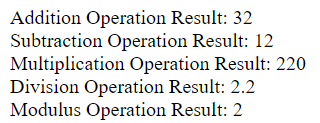# Arithmetic Operators

## Arithmetic Operators

Arithmetic operators are used to performing arithmetic operations on variables and data.

 Example Type Description \$a + \$b Addition Addition Sum of \$a and \$b. \$a - \$b Subtraction Difference of \$a and \$b. \$a * \$b Multiplication Product of \$a and \$b. \$a / \$b Division Quotient of \$a and \$b. \$a % \$b Modulo Remainder of \$a divided by \$b. \$a ** \$b Exponentiation Result of raising \$a to the \$b'th power.

Note: The division operator returns a float value unless the two operands are integers (or strings that get converted to integers) and the numbers are evenly divisible, in which case an integer value will be returned.

Example

<?PHP

\$a = 22;

\$b = 10;

\$sum = \$a + \$b;

echo "Addition Operation Result: \$sum <br/>";

\$sum = \$a - \$b;

echo "Subtraction Operation Result: \$sum <br/>";

\$sum = \$a * \$b;

echo "Multiplication Operation Result: \$sum <br/>";

\$sum = \$a / \$b;

echo "Division Operation Result: \$sum <br/>";

\$sum = \$a % \$b;

echo "Modulus Operation Result: \$sum <br/>";

?>

Result of the above example: